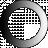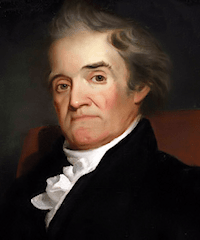Loading...

# Webster's Dictionary 1828## American DictionaryOF THEEnglish Language

Dictionary Search

### Theorem

THE'OREM, noun [Gr. to see.]

1. In mathematics, a proposition which terminates in theory, and which considers the properties of things already made or done; or it is a speculative proposition deduced from several definitions compared together.

A theorem is a proposition to be proved by a chain of reasoning. A theorem is something to be proved; a problem is something to be done.

2. In algebra or analysis, it is sometimes used to denote a rule, particularly when that rule is expressed by symbols.

A universal theorem extends to any quantity without restriction.

A particular theorem extends only to a particular quantity.

A negative theorem expresses the impossibility of any assertion.

A local theorem is that which relates to a surface.

A solid theorem is that which considers a space terminated by a solid, that is, by any of the three conic sections.